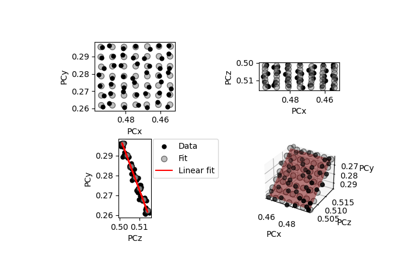# fit_pc#

EBSDDetector.fit_pc(pc_indices: , map_indices: , transformation: str = 'projective', is_outlier: = None, plot: bool = True, return_figure: bool = False, figure_kwargs: = None) [source]#

Return a new detector with interpolated projection centers (PCs) for all points in a map by fitting a plane to `pc` .

Parameters:
pc_indices

2D coordinates (row, column) of each `pc` in `map_coordinates`. Must be a flattened array of shape (2,) + `navigation_shape`.

map_indices

2D coordinates (row, column) of all map points in a regular grid to interpolate PCs for. Must be a flattened array of shape `(2,) + map_shape`.

transformation

Which transformation function to use when fitting PCs, either `"projective"` (default) or `"affine"`. Both transformations perserve co-planarity of map points, while the projective transformation allows parallel lines in the map point grid to become non-parallel within the sample plane.

is_outlier

Boolean array with `True` for PCs to not include in the fit. If not given, all PCs are used. Must be of `navigation_shape`.

plot

Whether to plot the experimental and estimated PCs (default is `True`).

return_figure

Whether to return the figure if `plot=True` (default is `False`).

figure_kwargs

Keyword arguments passed to `matplotlib.pyplot.Figure()` if `plot=True`.

Returns:
`new_detector`

New detector with as many interpolated PCs as indices given in `map_indices` and an estimated sample tilt. The detector tilt is assumed to be constant.

`fig`

Figure of experimental and estimated PCs, returned if `plot=True` and `return_figure=True`.

Raises:
`ValueError`

If `navigation_size` is 1 or if the `pc_indices` or `map_indices` arrays have the incorrect shape.

Notes

This method is adapted from Aimo Winkelmann’s functions `fit_affine()` and `fit_projective()` in the xcdskd Python package. Their uses are described in . Winkelmann refers to a code example from StackOverflow (https://stackoverflow.com/a/20555267/3228100) for the affine transformation.

## Examples using `EBSDDetector.fit_pc`#Fit a plane to selected projection centers

Fit a plane to selected projection centers